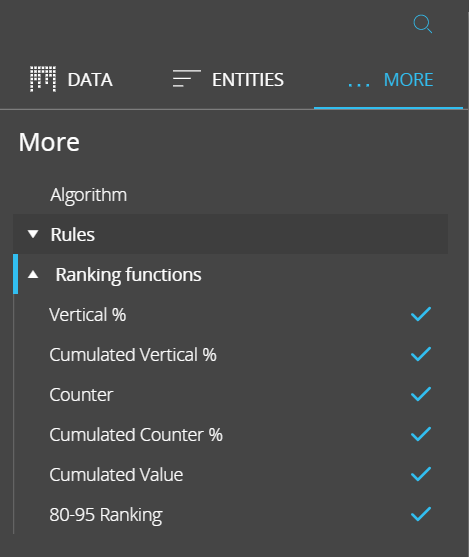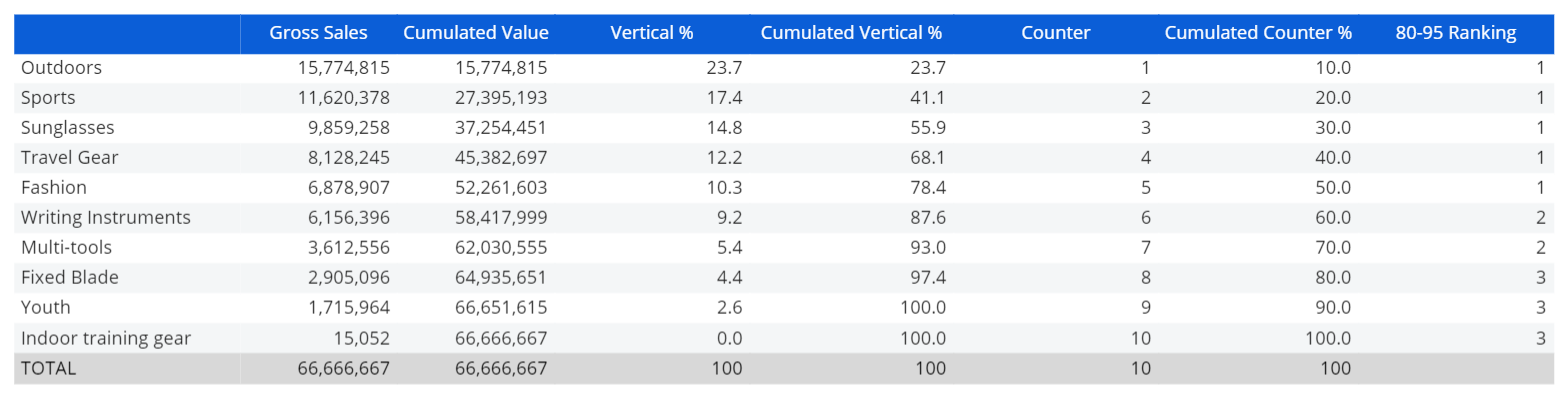# Ranking functions

Ranking functions are predefined functions which allow to quickly create ranking analyses. They're located in the Data panel of the Layout editor, in the "MORE" sub-page.

The selected function is always applied to the values of the previous Data Block in the list, taking into account only the most nested Entity set by row (i.e. the one in the rightmost position in the "BY ROW" field in the Axis area). Functions, therefore, cannot be the first Data Block in the list (Block "a") and in order for them to work, they need to be used in a Layout with at least one Entity set by row.## List of available ranking functions

• Vertical %. Returns the percentage value of the current row against the previous column total
• Cumulated Vertical %. Cumulates the Vertical% values progressively up to 100%. For best results, this function should be applied on sorted reports: go to the Filters tab to apply ascending or descending sorting based on values of a Data Block
• Counter. Row counter, counts rows progressively
• Cumulated Counter %. Returns the percentage represented by each row against the sum of all rows
• Cumulated Value. Cumulates values starting from the top row: each row is calculated by adding the value of the previous row to the value of the current row
• 80-95 Ranking. Returns 1, 2 or 3 based on the cumulated vertical % value of each row:
• if the value is less than 80%, it returns 1
• if it is between 80% and 95%, it returns 2
• if it is over 95%, it returns 3

For best results, this function should be applied on sorted reports: go to the Filters tab to apply ascending or descending sorting based on values of a Data Block.

## Example of an analysis using Ranking functionsThe Data View shown above has been obtained with the following Layout settings:

• Block "a" → Gross Sales cube: shows the total sales amount per product group, rows are sorted in descending order
• Block "b" → Cumulated Value: cumulates the sales values row by row
• Block "c" → Vertical %: returns the percentage value of each row against the Gross Sales column total value
• Block "d" → Cumulated Vertical %: returns the cumulated percentage of each row against the Gross Sales column total value
• Block "e" → Counter: counts the rows of the Layout.
• Block "f" → Cumulated Counter %: returns the cumulated percentage of the row counter
• Block "g" → 80-95 Ranking: returns 1 on the rows where the cumulated vertical % is lesser than or equal to 80% (this is the top tier), returns 2 on the rows where the cumulated vertical % is between 80% and 95% (this is the second tier of items) and returns 3 where the cumulated vertical % is above 95% (last tier).